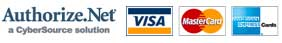A Family-owned Business

# Wire Size for LED Lights

Because wire has a certain amount of resistance, there will be a voltage drop as a result. For small gauges, long runs or relative high current loads, this voltage drop can become significant. The amount of light emitted from a LED is a function of the current going through it. As the voltage supplied drops, the amount of current going through the LED will drop as well. Since the LEDs are so efficient, it takes a significant drop of voltage to visibly effect the light output.

The Belden cables we sell have the following values of resistance: 22 gauge is 15 ohms/ 1000 feet and 20 gauge is 9.5 ohms/ 1000 feet. Bear in mind that a 100 foot wire run actually has a 200 foot path for the electrons.

Ohms Law: E=IxR where E is voltage measured in volts, I is current measured in amps and R is resistance measured in ohms.

For an example, what is the voltage drop in a 100 foot run of 22 gauge wire that feeds 10 Tri-Clusters or Micro Stars.

The resistance of the wire is 15/1000 X 200 = 3 ohms

The total current of 10 Tri-Clusters is 10 X .020 amps = .2 amps

The voltage drop is 3 X .2 = .6 volts

If the battery or power supply has a 13 volt output then the voltage at the Tri-Clusters is 13 - .6 = 12.4 volts. This is well within an acceptable range for the Tri-Clusters.

The Tri-Clusters and Micro Stars have been designed to work well with a voltage range of 11.6 to 14.6 volts.

The total current capacity of the 22-gauge wire is in excess of 2 amps and the capacity of the 20-gauge wire is in excess of 3 amps. This corresponds to a total of 100 or 300 lights respectively. Should lights in these quantities be used, we recommend using a larger gauge feed wire or multiple feeds from the power source.

In the example above, 100 lights would cause a voltage drop in 100 feet of 22-gauge wire of 6 volts. This would be unacceptable unless the voltage supplied was 18 volts. If the 20-gauge wire were used, you would see a voltage drop of 3.8 volts.

A reasonable rule of thumb is that the number of lights X the length of the wire run should be no greater than 1000 when using 22-gauge wire and it should be no greater than 1600 if using 20-gauge wire. This rule of thumb will allow for a maximum voltage drop of .6 volts.

These examples and figures are provided as a possible guide and are by no means absolute. Nothing can compare with empirical testing of the actual circuits at hand. The current drawn by the Tri-Clusters and Micro Stars actually varies with the voltage at the lights and as a function of temperature.\$30 minimum order required